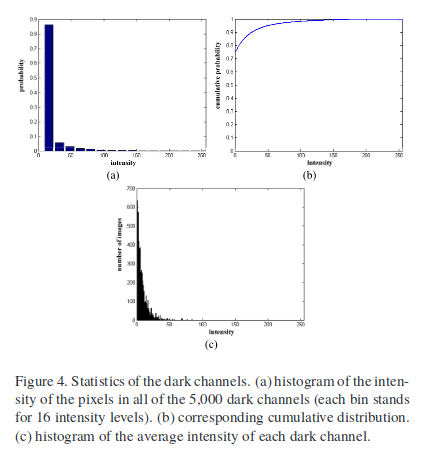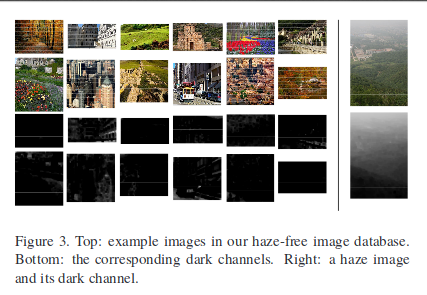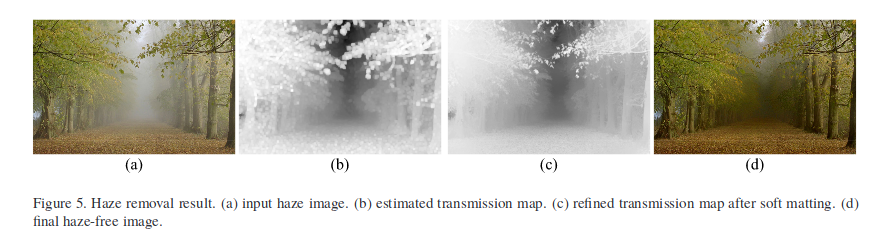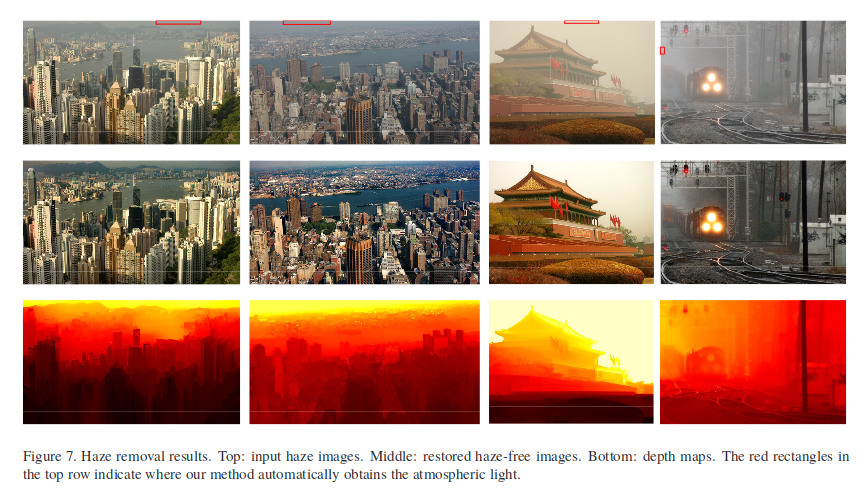### Basic Idea

$J^c$是一个室外图像$\mathbf J$的颜色通道（我们已经知道RGB图像有3个通道）, $\Omega (\mathbf{x})$是以$\mathbf x$为中心的一个图像区域。基于统计数据可以得到:$\min _{c}\left(\min _{\mathbf{y} \in \Omega(\mathbf{x})}\left(\frac{I^{c}(\mathbf{y})}{A^{c}}\right)\right)$正是经过normalize的雾气图$\frac{I^{c}(\mathbf{y})}{A^{c}}$的暗通道图，因此我们可以直接得到对于透射率的估计。在这里我们可以加另外一个参数$\omega$，因为透射率是1的情况很少，会使得照片显得不自然而且损失一些信息，因此我们可以通过调整$\omega$来使得结果更加自然，最终得到的透射率估计为:

### Soft Matting### A的估计